# NCERT Solutions For Class 10 Maths Chapter 14 Statistics Ex 14.3

Get Free NCERT Solutions for Class 10 Maths Chapter 14 Ex 14.3 PDF. Statistics Class 10 Maths NCERT Solutions are extremely helpful while doing your homework. Exercise 14.3 Class 10 Maths NCERT Solutions were prepared by Experienced ncert-books.in Teachers. Detailed answers of all the questions in Chapter 14 Maths Class 10 Statistics Exercise 14.3 provided in NCERT TextBook.

Question 1.
The following frequency distribution gives the monthly consumption of electricity of 68 consumers of a locality. Find the median, mean and mode of the data and compare them.Solution:Question 2.
If the median of the distribution given below is 28.5, find the values of x and y.Solution: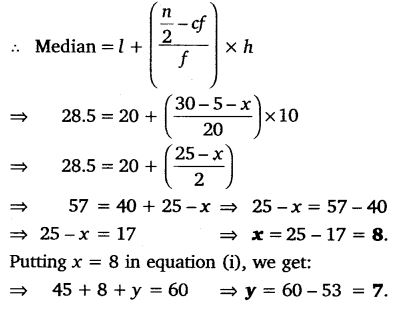Question 3.
A life insurance agent found the following data for distribution of ages of 100 policy holders. Calculate the median age, if policies are given only to persons having age 18 years onwards but less than 60 years.Solution: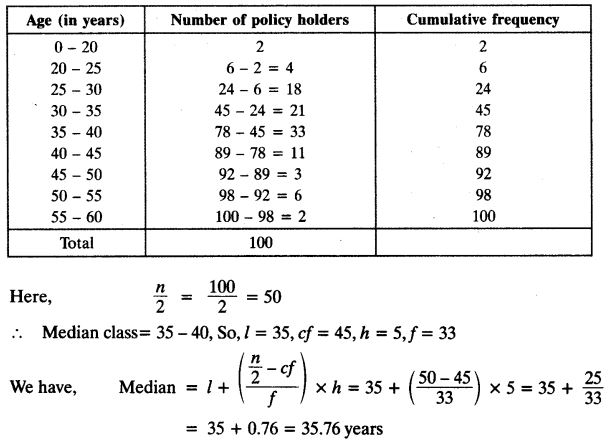Question 4.
The lengths of 40 leaves of a plant are measured correct to nearest millimetre, and the data obtained is represented in the following table:Find the median length of the leaves.
Solution: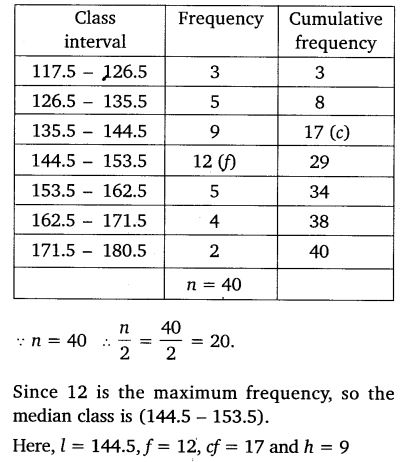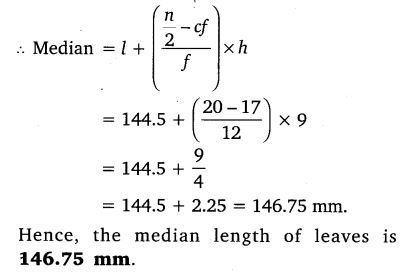Question 5.
The following table gives the distribution of the lifetime of 400 neon lamps:Find the median lifetime of a lamp.
Solution: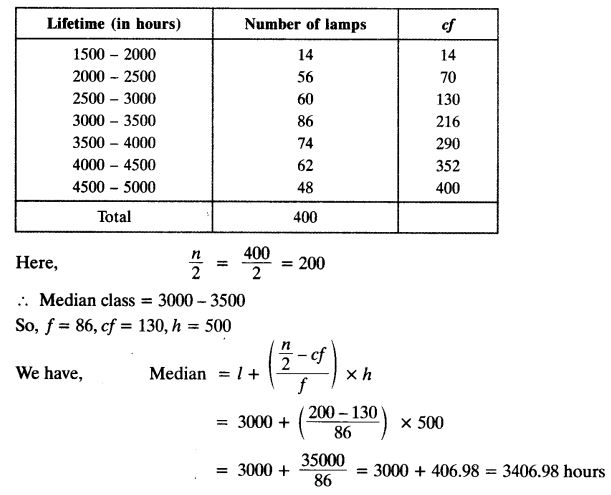Question 6.
100 surnames were randomly picked up from a local telephone directory and the frequency distribution of the number of letters in the English alphabet in the surnames was obtained as follows:Determine the median number of letters in the surnames. Find the mean number of letters in the surnames. Also, find the modal size of the surnames.
Solution: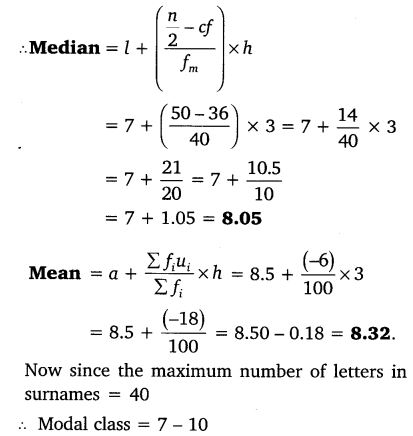Question 7.
The distribution below gives the weight of 30 students of a class. Find the median weight of the students.Solution: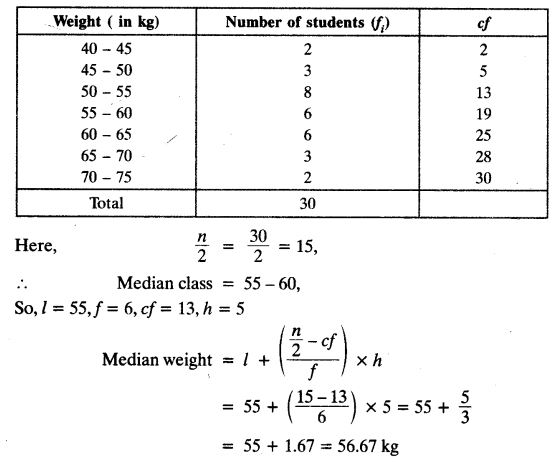+